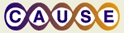# Using an Applet to Demonstrate Confidence IntervalsThis material was originally developed through CAUSE
as part of its collaboration with the SERC Pedagogic Service.

#### Summary

Students work individually with an applet to enhance their understanding of confidence intervals. Using a detailed step by step activity, they will use simulated data from the applet to determine the percentage of confidence intervals that capture the population proportion.

## Learning Goals

Students should be able to:
• Explain that confidence intervals are random quantities which vary from sample to sample and that they may miss the true population parameter.
• Explain that the confidence level is that proportion of possible samples for which the confidence interval will capture the true parameter.
• Given a study and confidence interval for the population proportion, describe how the following will affect the width of the confidence interval.
• Increasing the sample size
• Increasing the confidence level C

## Context for Use

This activity is appropriate for a college introductory statistics course for any class size. This can be done in a lab setting or as an out-of-class assignment.
Before beginning, students need to be familiar with
• Given a confidence level C, determine the critical value (z*) from the standard normal table needed to construct the confidence interval.
• Construct a confidence interval for a population proportion using the formula.

## Description and Teaching Materials

Student Handout (Microsoft Word 141kB Aug14 06)

## Teaching Notes and Tips

• It is very likely students will have different % coverage between their simulations of n=50 and n=100. Make sure to emphasize in class that this is due to sampling variability and not a change in the confidence level.
• Be sure to have students bring in their handout with results so that you can follow-up with a compilation of results.

## Assessment

Make sure to emphasize in class the following day:
• When the sample size changes from 50 to 100 the confidence level ________. From this we can conclude that the sample size _________ the confidence level. This way the students understand what they are expected to learn from the assignment.
• An optional addition to the in class discussion is to suggest to your students to go back and run a simulation of 1000 samples or higher so that they see that percent coverage settles around 95%.

## References and Resources

http://www.stat.vt.edu/~sundar/java/applets/CI.html